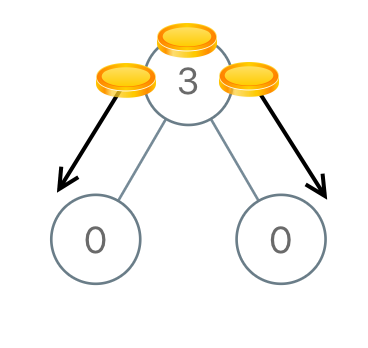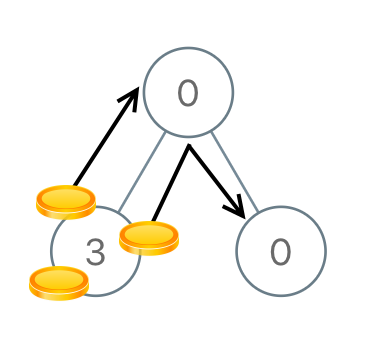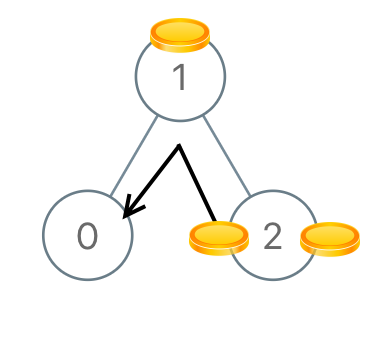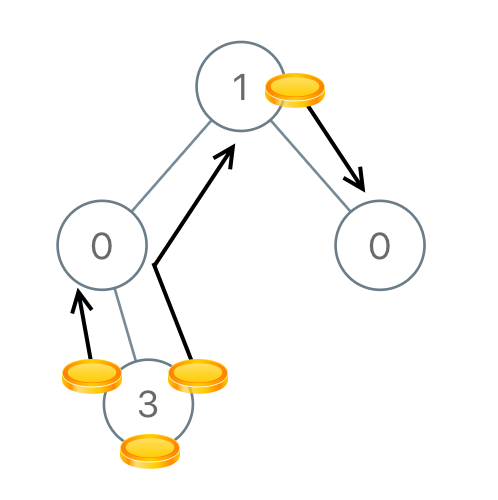##### Welcome to Subscribe On Youtube

Formatted question description: https://leetcode.ca/all/979.html

# 979. Distribute Coins in Binary Tree (Medium)

Given the root of a binary tree with N nodes, each node in the tree has node.val coins, and there are N coins total.

In one move, we may choose two adjacent nodes and move one coin from one node to another.  (The move may be from parent to child, or from child to parent.)

Return the number of moves required to make every node have exactly one coin.

Example 1:Input: [3,0,0]
Output: 2
Explanation: From the root of the tree, we move one coin to its left child, and one coin to its right child.


Example 2:Input: [0,3,0]
Output: 3
Explanation: From the left child of the root, we move two coins to the root [taking two moves].  Then, we move one coin from the root of the tree to the right child.


Example 3:Input: [1,0,2]
Output: 2


Example 4:Input: [1,0,0,null,3]
Output: 4


Note:

1. 1<= N <= 100
2. 0 <= node.val <= N

Companies:

Related Topics:
Tree, Depth-first Search

Similar Questions:

## Solution 1.

// OJ: https://leetcode.com/problems/distribute-coins-in-binary-tree/
// Time: O(N)
// Space: O(H)
class Solution {
private:
int ans = 0;
int postorder(TreeNode *root) {
if (!root) return 0;
int move = 1 - root->val + postorder(root->left) + postorder(root->right);
ans += abs(move);
return move;
}
public:
int distributeCoins(TreeNode* root) {
postorder(root);
return ans;
}
};


Java

• /**
* Definition for a binary tree node.
* public class TreeNode {
*     int val;
*     TreeNode left;
*     TreeNode right;
*     TreeNode(int x) { val = x; }
* }
*/
class Solution {
public int distributeCoins(TreeNode root) {
int moves = 0;
List<TreeNode> list = new ArrayList<TreeNode>();
queue.offer(root);
while (!queue.isEmpty()) {
TreeNode node = queue.poll();
TreeNode left = node.left, right = node.right;
if (left != null)
queue.offer(left);
if (right != null)
queue.offer(right);
}
for (int i = list.size() - 1; i >= 0; i--) {
TreeNode node = list.get(i);
TreeNode left = node.left, right = node.right;
if (left != null) {
int leftVal = left.val;
int difference = leftVal - 1;
left.val -= difference;
node.val += difference;
moves += Math.abs(difference);
}
if (right != null) {
int rightVal = right.val;
int difference = rightVal - 1;
right.val -= difference;
node.val += difference;
moves += Math.abs(difference);
}
}
return moves;
}
}

• // OJ: https://leetcode.com/problems/distribute-coins-in-binary-tree/
// Time: O(N)
// Space: O(H)
class Solution {
private:
int ans = 0;
int postorder(TreeNode *root) {
if (!root) return 0;
int move = 1 - root->val + postorder(root->left) + postorder(root->right);
ans += abs(move);
return move;
}
public:
int distributeCoins(TreeNode* root) {
postorder(root);
return ans;
}
};

• # Definition for a binary tree node.
# class TreeNode:
#     def __init__(self, val=0, left=None, right=None):
#         self.val = val
#         self.left = left
#         self.right = right
class Solution:
def distributeCoins(self, root: TreeNode) -> int:
def dfs(root):
nonlocal ans
if root is None:
return 0
left, right = dfs(root.left), dfs(root.right)
ans += abs(left) + abs(right)
return left + right + root.val - 1

ans = 0
dfs(root)
return ans

############

# 979. Distribute Coins in Binary Tree
# https://leetcode.com/problems/distribute-coins-in-binary-tree/

# Definition for a binary tree node.
# class TreeNode:
#     def __init__(self, val=0, left=None, right=None):
#         self.val = val
#         self.left = left
#         self.right = right
class Solution:
def distributeCoins(self, root: Optional[TreeNode]) -> int:
res = 0

def go(node):
nonlocal res

if not node: return 0

left, right = go(node.left), go(node.right)
coins = left + right + node.val
res += abs(coins - 1)
return coins - 1

go(root)
return res